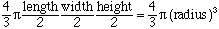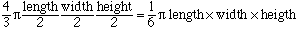Sender: Michael Nowicki I am a physician at the University of Mississippi. I am trying to compare the size of polyps (abnormal growth of tissue in the colon) between 2 groups of individuals. The department of pathology gives the size as length X width X depth - how do I calculate the volume? Polyps are nearly ovoid in shape. I appreciate any help you can provide. Sincerely: Michael Nowicki Michael, If it is 'oval' meaning ellipsoid, then the appropriate volume formula is a modification of the volume of a sphere. In the terms you have been given, the volume of a sphere iswhere all three measurements happen to be equal. For the ellisoid (oval) you stretch the 'length' - and that stretches the volume by the same ratio, then the width - and that also stretches the volume by the second ratio.    The net result is STILL the formulaWalter Go to Math Central